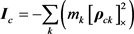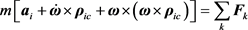# 刚体空间运动的动力学方程的联合推导United Derivation of Dynamical Equations for Rigid Body Spatial Motion

• 全文下载: PDF(498KB)    PP.197-203   DOI: 10.12677/IJM.2019.83022
• 下载量: 228  浏览量: 426

Dynamical equations for rigid body spatial motion are derived from Dynamical equations of system of particles, which is a more fundamental theoretical basis than the momentum theorem and angular momentum theorem. Linear acceleration and angular acceleration are coupled with each other in the present dynamical equations which lead to solve the equation is extremely difficult, especially under conditions of high speed rotation. The derivation coupled the translational for-mulation and the rotational formulation, and uncoupled the linear acceleration and angular ac-celeration. Based on the derivation process, the relationship between the equations and the exist-ing forms is discussed; the main differences from the existing derivation process are stated.

1. 引言

2. 质点系动力学方程概要

${\stackrel{˙}{r}}_{ok}={v}_{k}$ (1)

${\stackrel{˙}{v}}_{k}={a}_{k}$ (2)

$\underset{k}{\sum }{m}_{k}{a}_{k}=\underset{k}{\sum }{F}_{k}$ (3)

$\underset{k}{\sum }\left({r}_{ok}×{m}_{k}{a}_{k}\right)=\underset{k}{\sum }\left({r}_{ok}×{F}_{k}\right)$ (4)

$m{r}_{oc}=\underset{k}{\sum }{m}_{k}{r}_{ok}$ (5)

$m=\underset{k}{\sum }{m}_{k}$ (6)

3. 刚体动力学方程

3.1. 质点位置的分解

${r}_{ok}={r}_{oi}+{\rho }_{ik}$ (7)

${\rho }_{ik}={\rho }_{ic}+{\rho }_{ck}$ (8)

${r}_{ok}={r}_{oi}+{\rho }_{ic}+{\rho }_{ck}$ (9)

$\underset{k}{\sum }{m}_{k}{\rho }_{ck}=0$ (10)(11)

3.2. 质点速度与加速度的分解

$\begin{array}{l}{\stackrel{˙}{\rho }}_{ik}=\omega ×{\rho }_{ik}\\ {\stackrel{˙}{\rho }}_{ic}=\omega ×{\rho }_{ic}\\ {\stackrel{˙}{\rho }}_{ck}=\omega ×{\rho }_{ck}\end{array}\right\}$ (12)

${v}_{k}={v}_{i}+\omega ×\left({\rho }_{ic}+{\rho }_{ck}\right)$ (13)

${a}_{k}={a}_{i}+\stackrel{˙}{\omega }×\left({\rho }_{ic}+{\rho }_{ck}\right)+\omega ×\left[\omega ×\left({\rho }_{ic}+{\rho }_{ck}\right)\right]$ (14)

${a}_{c}={a}_{i}+\stackrel{˙}{\omega }×{\rho }_{ic}+\omega ×\left(\omega ×{\rho }_{ic}\right)$ (15)

3.3. 刚体动力学方程推导

$\underset{k}{\sum }\left[\left({r}_{oi}+{\rho }_{ic}+{\rho }_{ck}\right)×{m}_{k}{a}_{k}\right]=\underset{k}{\sum }\left[\left({r}_{oi}+{\rho }_{ic}+{\rho }_{ck}\right)×{F}_{k}\right]$ (16)

$\begin{array}{l}{r}_{oi}×\left(\underset{k}{\sum }{m}_{k}{a}_{k}\right)+{\rho }_{ic}×\left(\underset{k}{\sum }{m}_{k}{a}_{k}\right)+\underset{k}{\sum }\left({\rho }_{ck}×{m}_{k}{a}_{k}\right)\\ ={r}_{oi}×\left(\underset{k}{\sum }{F}_{k}\right)+{\rho }_{ic}×\left(\underset{k}{\sum }{F}_{k}\right)+\underset{k}{\sum }\left({\rho }_{ck}×{F}_{k}\right)\end{array}$ (17)

${r}_{oi}×\left(\underset{k}{\sum }{m}_{k}{a}_{k}\right)={r}_{oi}×\left(\underset{k}{\sum }{F}_{k}\right)$ (18)

${\rho }_{ic}×\left(\underset{k}{\sum }{m}_{k}{a}_{k}\right)={\rho }_{ic}×\left(\underset{k}{\sum }{F}_{k}\right)$ (19)

$\underset{k}{\sum }\left({\rho }_{ck}×{m}_{k}{a}_{k}\right)=\underset{k}{\sum }\left({\rho }_{ck}×{F}_{k}\right)$ (20)

$\underset{k}{\sum }{m}_{k}\left\{{a}_{i}+\stackrel{˙}{\omega }×\left({\rho }_{ic}+{\rho }_{ck}\right)+\omega ×\left[\omega ×\left({\rho }_{ic}+{\rho }_{ck}\right)\right]\right\}=\underset{k}{\sum }{F}_{k}$ (21)

$\begin{array}{l}\left(\underset{k}{\sum }{m}_{k}\right)\left[{a}_{i}+\stackrel{˙}{\omega }×{\rho }_{ic}+\omega ×\left(\omega ×{\rho }_{ic}\right)\right]\\ \text{ }+\stackrel{˙}{\omega }×\left(\underset{k}{\sum }{m}_{k}{\rho }_{ck}\right)+\omega ×\left[\omega ×\left(\underset{k}{\sum }{m}_{k}{\rho }_{ck}\right)\right]=\underset{k}{\sum }{F}_{k}\end{array}$ (22)(23)

$\underset{k}{\sum }\left({\rho }_{ck}×{m}_{k}\left({a}_{i}+\stackrel{˙}{\omega }×\left({\rho }_{ic}+{\rho }_{ck}\right)+\omega ×\left(\omega ×\left({\rho }_{ic}+{\rho }_{ck}\right)\right)\right)\right)=\underset{k}{\sum }\left({\rho }_{ck}×{F}_{k}\right)$ (24)

$\begin{array}{l}\left(\underset{k}{\sum }{m}_{k}{\rho }_{ck}\right)×\left({a}_{i}+\stackrel{˙}{\omega }×{\rho }_{ic}+\omega ×\left(\omega ×{\rho }_{ic}\right)\right)\\ \text{ }+\underset{k}{\sum }\left({m}_{k}{\rho }_{ck}×\left(\stackrel{˙}{\omega }×{\rho }_{ck}\right)\right)+\underset{k}{\sum }\left({m}_{k}{\rho }_{ck}×\left(\omega ×\left(\omega ×{\rho }_{ck}\right)\right)\right)\\ =\underset{k}{\sum }\left({\rho }_{ck}×{F}_{k}\right)\end{array}$ (25)

$\underset{k}{\sum }\left({m}_{k}{\rho }_{ck}×\left(\stackrel{˙}{\omega }×{\rho }_{ck}\right)\right)={I}_{c}\stackrel{˙}{\omega }$ (26)(27)

 (28)

${I}_{c}\stackrel{˙}{\omega }+\omega ×\left({I}_{c}\omega \right)=\underset{k}{\sum }\left({\rho }_{ck}×{F}_{k}\right)$ (29)

$m\left[{a}_{i}+\stackrel{˙}{\omega }×{\rho }_{ic}+\omega ×\left(\omega ×{\rho }_{ic}\right)\right]=\underset{k}{\sum }{F}_{k}$ (30a)

${I}_{c}\stackrel{˙}{\omega }+\omega ×\left({I}_{c}\omega \right)=\underset{k}{\sum }\left({\rho }_{ck}×{F}_{k}\right)$ (30b)

${a}_{c}={a}_{i}+\stackrel{˙}{\omega }×{\rho }_{ic}+\omega ×\left(\omega ×{\rho }_{ic}\right)$ (31a)

$m{a}_{c}=\underset{k}{\sum }{F}_{k}$ (31b)

${I}_{c}\stackrel{˙}{\omega }+\omega ×\left({I}_{c}\omega \right)=\underset{k}{\sum }\left({\rho }_{ck}×{F}_{k}\right)$ (31c)

4. 讨论

4.1. 与现有方程的关系

${\rho }_{ic}×m{a}_{i}+{I}_{i}\stackrel{˙}{\omega }+\omega ×\left({I}_{i}\omega \right)={\rho }_{ic}×\left(\underset{k}{\sum }{F}_{k}\right)+\underset{k}{\sum }\left({\rho }_{ck}×{F}_{k}\right)$ (32)

${I}_{i}=-m{\left[{\rho }_{ic}\right]}_{×}^{2}+{I}_{c}$ (33)

$\begin{array}{l}{\rho }_{ic}×m{a}_{i}+\left({I}_{c}-m{\left[{\rho }_{ic}\right]}_{×}^{2}\right)\stackrel{˙}{\omega }+\omega ×\left(\left({I}_{c}-m{\left[{\rho }_{ic}\right]}_{×}^{2}\right)\omega \right)\\ ={\rho }_{ic}×\left(\underset{k}{\sum }{F}_{k}\right)+\underset{k}{\sum }\left({\rho }_{ck}×{F}_{k}\right)\end{array}$ (34)

$\begin{array}{l}{\rho }_{ic}×m{a}_{i}-m{\left[{\rho }_{ic}\right]}_{×}^{2}\stackrel{˙}{\omega }-m\omega ×\left({\left[{\rho }_{ic}\right]}_{×}^{2}\omega \right)+{I}_{c}\stackrel{˙}{\omega }+\omega ×\left({I}_{c}\omega \right)\\ ={\rho }_{ic}×\left(\underset{k}{\sum }{F}_{k}\right)+\underset{k}{\sum }\left({\rho }_{ck}×{F}_{k}\right)\end{array}$ (35)

$\begin{array}{l}{\rho }_{ic}×m{a}_{i}-m{\left[{\rho }_{ic}\right]}_{×}^{2}\stackrel{˙}{\omega }-m\omega ×\left({\left[{\rho }_{ic}\right]}_{×}^{2}\omega \right)\\ ={\rho }_{ic}×m\left[{a}_{i}+\stackrel{˙}{\omega }×{\rho }_{ic}+\omega ×\left(\omega ×{\rho }_{ic}\right)\right]\end{array}$ (36)

${\rho }_{ic}×m\left[{a}_{i}+\stackrel{˙}{\omega }×{\rho }_{ic}+\omega ×\left(\omega ×{\rho }_{ic}\right)\right]={\rho }_{ic}×\left(\underset{k}{\sum }{F}_{k}\right)$ (37)

${I}_{c}\stackrel{˙}{\omega }+\omega ×\left({I}_{c}\omega \right)=\underset{k}{\sum }\left({\rho }_{ck}×{F}_{k}\right)$ (38)

4.2. 与现有推导的主要差异

4.3. 刚体一般运动的表述方式

5. 结束语

  洪嘉振. 关于刚体平面运动动力学方程——理论力学若干概念的思考[J]. 力学与实践, 2015, 37(6): 731-736.  沈惠川, 李书民. 经典力学[M]. 合肥: 中国科学技术大学出版社, 2006.  刘延柱, 潘振宽, 戈新生. 多体系统动力学[M]. 第二版. 北京: 高等教育出版社, 2014.  洪嘉振. 计算多体系统动力学[M]. 北京: 高等教育出版社, 1999.  Wittenburg, J. (2008) Dynamics of Multibody Systems. Springer, Berlin Heidelberg.  Featherstone, R. (2008) Rigid Body Dynamics Algorithms. Springer US, Boston. https://doi.org/10.1007/978-1-4899-7560-7  李洲圣. 陀螺动力学仿真研究,翻身陀螺[J]. 软件, 2014, 35(5): 73-84.  Eberhard, P. and Schiehlen, W. (2005) Computational Dynamics of Multibody Systems: History, Formalisms, and Applications. Journal of Computational and Nonlinear Dynamics, 1, 3-12. https://doi.org/10.1115/1.1961875  Schiehlen, W. (1997) Multibody System Dynamics: Roots and Perspectives. Multibody System Dynamics, 1, 149-188. https://doi.org/10.1023/A:1009745432698  Hibbeler, R.C. 动力学[M]. 李俊峰, 袁长清, 吕敬, 译. 北京: 机械工业出版社, 2014.  张戡. 平面运动刚体的角速度与基点选择无关的证明[J]. 大学物理, 1982, 1(12): 4-5.  秦家桦. 对“平面运动的刚体的角速度与基点选择无关的证明”一文的补充[J]. 大学物理, 1984, 3(3): 51-27.  王希凡. 刚体角速度和角加速度与基点选择无关的证明[J]. 大学物理, 1990, 9(12): 43.  张明影. 关于刚体转动的角速度和角加速度的讨论[J]. 西安航空技术高等专科学校学报, 2002, 20(1): 49-50.